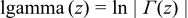#Boost C++ Libraries

...one of the most highly regarded and expertly designed C++ library projects in the world.

This is the documentation for an old version of boost. Click here for the latest Boost documentation.

### C99 C Functions

##### Supported C99 Functions
```namespace boost{ namespace math{ namespace tr1{ extern "C"{

typedef unspecified float_t;
typedef unspecified double_t;

double acosh(double x);
float acoshf(float x);
long double acoshl(long double x);

double asinh(double x);
float asinhf(float x);
long double asinhl(long double x);

double atanh(double x);
float atanhf(float x);
long double atanhl(long double x);

double cbrt(double x);
float cbrtf(float x);
long double cbrtl(long double x);

double copysign(double x, double y);
float copysignf(float x, float y);
long double copysignl(long double x, long double y);

double erf(double x);
float erff(float x);
long double erfl(long double x);

double erfc(double x);
float erfcf(float x);
long double erfcl(long double x);

double expm1(double x);
float expm1f(float x);
long double expm1l(long double x);

double fmax(double x, double y);
float fmaxf(float x, float y);
long double fmaxl(long double x, long double y);

double fmin(double x, double y);
float fminf(float x, float y);
long double fminl(long double x, long double y);

double hypot(double x, double y);
float hypotf(float x, float y);
long double hypotl(long double x, long double y);

double lgamma(double x);
float lgammaf(float x);
long double lgammal(long double x);

long long llround(double x);
long long llroundf(float x);
long long llroundl(long double x);

double log1p(double x);
float log1pf(float x);
long double log1pl(long double x);

long lround(double x);
long lroundf(float x);
long lroundl(long double x);

double nextafter(double x, double y);
float nextafterf(float x, float y);
long double nextafterl(long double x, long double y);

double nexttoward(double x, long double y);
float nexttowardf(float x, long double y);
long double nexttowardl(long double x, long double y);

double round(double x);
float roundf(float x);
long double roundl(long double x);

double tgamma(double x);
float tgammaf(float x);
long double tgammal(long double x);

double trunc(double x);
float truncf(float x);
long double truncl(long double x);

}}}} // namespaces
```

In addition sufficient additional overloads of the `double` versions of the above functions are provided, so that calling the function with any mixture of `float`, `double`, ```long double```, or integer arguments is supported, with the return type determined by the result type calculation rules.

For example:

```acoshf(2.0f);  // float version, returns float.
acosh(2.0f);   // also calls the float version and returns float.
acosh(2.0);    // double version, returns double.
acoshl(2.0L);  // long double version, returns a long double.
acosh(2.0L);   // also calls the long double version.
acosh(2);      // integer argument is treated as a double, returns double.
```
##### Quick Reference

More detailed descriptions of these functions are available in the C99 standard.

```typedef unspecified float_t;
typedef unspecified double_t;
```

In this implementation `float_t` is the same as type `float`, and `double_t` the same as type `double` unless the preprocessor symbol FLT_EVAL_METHOD is defined, in which case these are set as follows:

FLT_EVAL_METHOD

float_t

double_t

0

float

double

1

double

double

2

long double

long double

```double acosh(double x);
float acoshf(float x);
long double acoshl(long double x);
```

Returns the inverse hyperbolic cosine of x.

```double asinh(double x);
float asinhf(float x);
long double asinhl(long double x);
```

Returns the inverse hyperbolic sine of x.

```double atanh(double x);
float atanhf(float x);
long double atanhl(long double x);
```

Returns the inverse hyperbolic tangent of x.

```double cbrt(double x);
float cbrtf(float x);
long double cbrtl(long double x);
```

Returns the cubed root of x.

```double copysign(double x, double y);
float copysignf(float x, float y);
long double copysignl(long double x, long double y);
```

Returns a value with the magnitude of x and the sign of y.

```double erf(double x);
float erff(float x);
long double erfl(long double x);
```

Returns the error function of x:```double erfc(double x);
float erfcf(float x);
long double erfcl(long double x);
```

Returns the complementary error function of x `1-erf(x)` without the loss of precision implied by the subtraction.

```double expm1(double x);
float expm1f(float x);
long double expm1l(long double x);
```

Returns `exp(x)-1` without the loss of precision implied by the subtraction.

```double fmax(double x, double y);
float fmaxf(float x, float y);
long double fmaxl(long double x, long double y);
```

Returns the larger (most positive) of x and y.

```double fmin(double x, double y);
float fminf(float x, float y);
long double fminl(long double x, long double y);
```

Returns the smaller (most negative) of x and y.

```double hypot(double x, double y);
float hypotf(float x, float y);
long double hypotl(long double x, long double y);
```

Returns ```sqrt(x*x + y*y)``` without the danger of numeric overflow implied by that formulation.

```double lgamma(double x);
float lgammaf(float x);
long double lgammal(long double x);
```

Returns the log of the gamma function of x.```long long llround(double x);
long long llroundf(float x);
long long llroundl(long double x);
```

Returns the value x rounded to the nearest integer as a `long long`: equivalent to `floor(x + 0.5)`

```double log1p(double x);
float log1pf(float x);
long double log1pl(long double x);
```

Returns the `log(x+1)` without the loss of precision implied by that formulation.

```long lround(double x);
long lroundf(float x);
long lroundl(long double x);
```

Returns the value x rounded to the nearest integer as a `long`: equivalent to `floor(x + 0.5)`

```double nextafter(double x, double y);
float nextafterf(float x, float y);
long double nextafterl(long double x, long double y);
```

Returns the next representable floating point number after x in the direction of y, or x if ```x == y```.

```double nexttoward(double x, long double y);
float nexttowardf(float x, long double y);
long double nexttowardl(long double x, long double y);
```

As `nextafter`, but with y always expressed as a `long double`.

```double round(double x);
float roundf(float x);
long double roundl(long double x);
```

Returns the value x rounded to the nearest integer: equivalent to `floor(x + 0.5)`

```double tgamma(double x);
float tgammaf(float x);
long double tgammal(long double x);
```

Returns the gamma function of x:```double trunc(double x);